• SSIM的全称为structural similarity index，即为结构相似性，是一种衡量两幅图像相似度的指标。该指标首先由德州大学奥斯丁分校的图像和视频工程实验室(Laboratory for Image and Video Engineering)提出。而如果...
SSIM的全称为structural similarity index，即为结构相似性，是一种衡量两幅图像相似度的指标。该指标首先由德州大学奥斯丁分校的图像和视频工程实验室(Laboratory for Image and Video Engineering)提出。而如果两幅图像是压缩前和压缩后的图像，那么SSIM算法就可以用来评估压缩后的图像质量。
SSIM如何表征相似性：  先给出一组公式：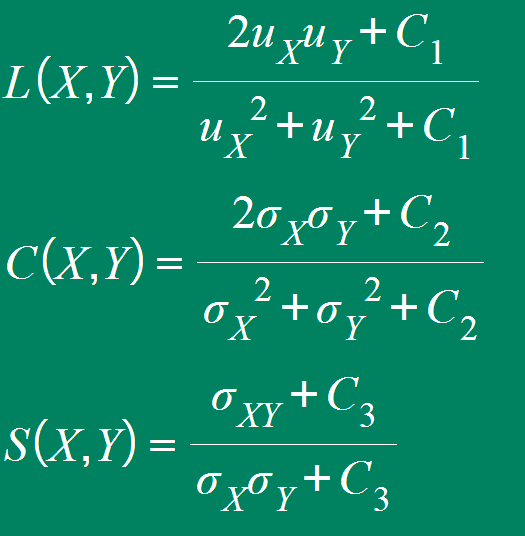uX、uY分别表示图像X和Y的均值，σX、σY分别表示图像X和Y的标准差，σX*σX、σY*σY（实在打不出上标啊，理解万岁）分别表示图像X和Y的方差。σXY代表图像X和Y协方差。C1，C2和C3为常数，是为了避免分母为0而维持稳定。通常取C1=(K1*L)^2, C2=(K2*L)^2, C3=C2/2, 一般地K1=0.01, K2=0.03, L=255（ 是像素值的动态范围，一般都取为255）
最后的SSIM指数为：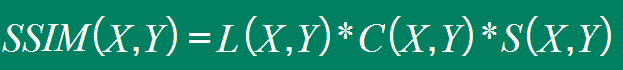当我们设定C3=C2/2时，我们可以将公式改写成更加简单的形式：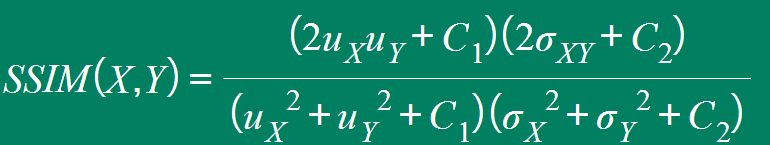所以结构相似度指数从图像组成的角度将结构信息定义为独立于亮度、对比度的反映场景中物体结构的属性，并将失真建模为亮度、对比度和结构三个不同因素的组合。用均值作为亮度的估计，标准差作为对比度的估计，协方差作为结构相似程度的度量。
如何求得均值，方差与标准差：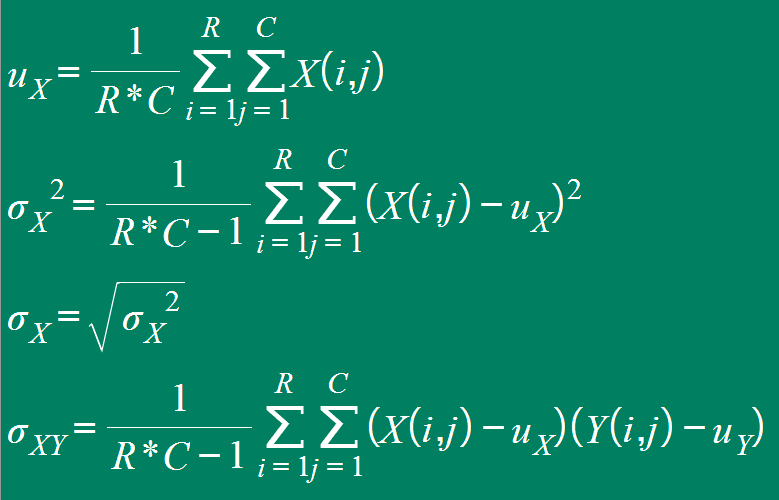而在实际应用中，一般采用高斯函数计算图像的均值、方差以及协方差，而不是采用遍历像素点的方式，以换来更高的效率。
下面的链接我们将用一个简单的程序实现SSIM算法，并作出对比实验：  http://blog.csdn.net/chaipp0607/article/details/70160307
展开全文SSIM
• 图像质量评估算法,结构相似性(SSIM)，matlab代码（亲测可使用），用于图像质量评价，原始图像与处理后的图像计算它们的峰值信噪比，比值越高越优。 SSIM 公式基于样本 x 和 y 之间的三个比较衡量：亮度 (luminance)...
• 我们提出了一种基于结构相似性和视觉掩蔽的改进的客观图像质量评估方法，称为感知图像质量评估（PIQA）。 PIQA包含三个相似性度量：亮度比较度量，结构比较度量，与结构相似性（SSIM）相同的对比度比较度量及其变体...
• 结构相似性指数测量（SSIM）是全参考图像质量评估指标（FR-IQA）中最著名的方法之一。 为了提高SSIM的性能，本文提出了一种基于谐波均值的池化策略。 提出的用谐波均值代替算术平均值的方法倾向于在使用倒数变换的...
• 图像质量评估：从误差的能见性到结构相似性.doc文档
• 前一篇文章参考：图像质量评估各项指标（一） 一、从均方误差MSE和峰值信噪比PSNR说起 计算图像降噪后的质量，最 直接的思路即比较降噪后的图像与真实图像（distortion-free）之间的差剖面，即可视误差，通过 v.....前言：前面的一片文章中较为系统的归纳了图像质量评价的各种指标，其中使用的最多的就是PSNR和SSIM，鉴于PSNR较为简单，本文专门介绍SSIM的思想以及它的实现。
前一篇文章参考：图像质量评估各项指标（一）
一、从均方误差MSE和峰值信噪比PSNR说起
计算图像降噪后的质量，最 直接的思路即比较降噪后的图像与真实图像（distortion-free）之间的差剖面，即可视误差，通过 visibility of errors 评价图像质量。PSNR 和 MSE 就是基于这种简单直接的思路确定的指标，
MSE（Mean Squared Error），顾名思义，定义略。
PSNR（Peak Signal to Noise Ratio），峰值信噪比，即峰值信号的能量与噪声的平均能量之比，通常表示的时候取 log 变成分贝（dB），由于 MSE 为真实图像与含噪图像之差的能量均值，而两者的差即为噪声，因此 PSNR 即峰值信号能量与 MSE 之比。定义式如下：​    第二个等式由于图像像素点数值以量化方式保存，bits 即每个像素点存储所占的位数。因此 MaxValue 即为 2^bits - 1。
计算PSNR
def cal_psnr(im1, im2):
mse = (np.abs(im1 - im2) ** 2).mean()
psnr = 10 * np.log10(255 * 255 / mse)
return psnr
由于 灰度等级gray scale 存成 8bit ，故最大值255。
当时上面的评价指标有一个大的问题，那就是 由于基于差剖面的简单计算不符合人类视觉系统（Human Visual System，HVS）的评价结果，因此需要对评价方式进行重新考量。如果图片的最终目的是对人类展示的话，那么质量应该以人的主管测评为准。但是由于主管评价不方便且费时，因此我们试图用客观的 图像质量评价来对图像进行评价，使其接近 HVS 的特点。由于 HVS 具有可以抓取图像的结构特征的特点，因此设计（结构相似性） Structural Similarity 进行评价，即 SSIM 。
注意：PSNR和SSIM都是全参考的（full reference）的，也就是需要无噪声的真实图像作为参考依据。

二、结构相似性SSIM
SSIM的基本思路是，通过以下三个方面来对两幅图像的相似性进行评估，即

1. luminance，亮度 2. contrast，对比度 3. structure，结构

算法的框图基本如下：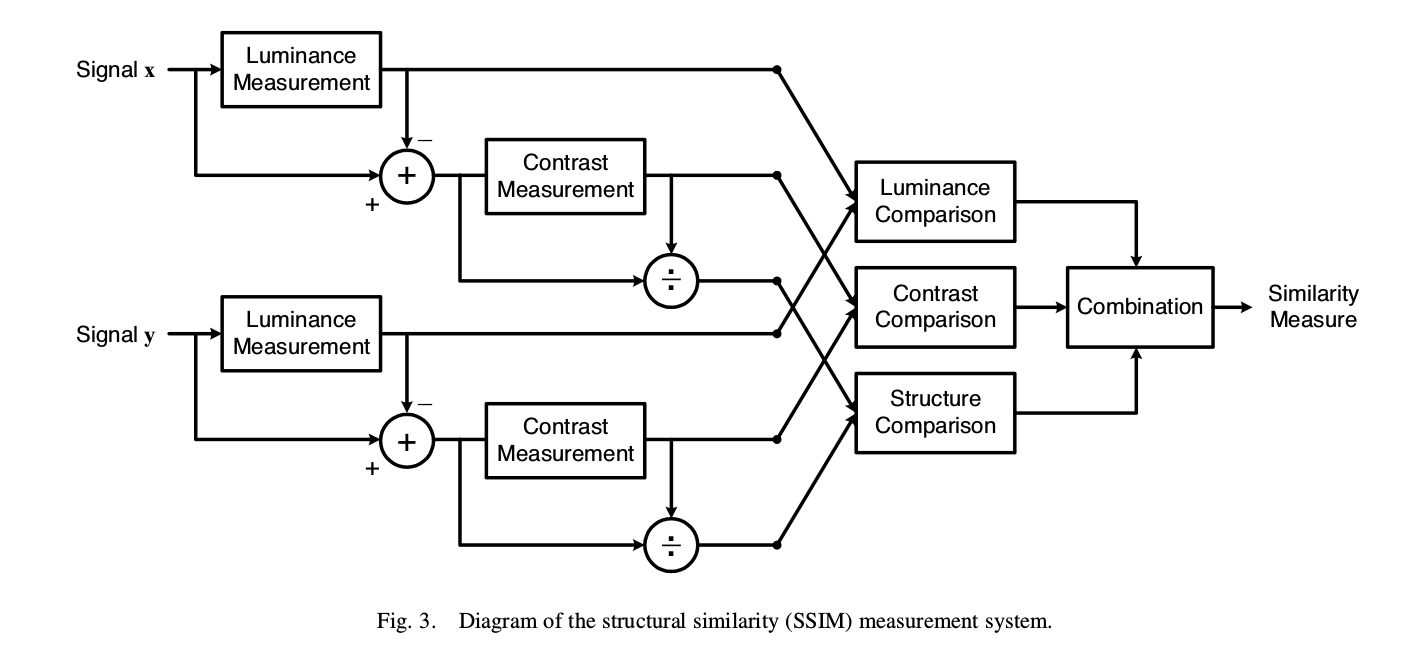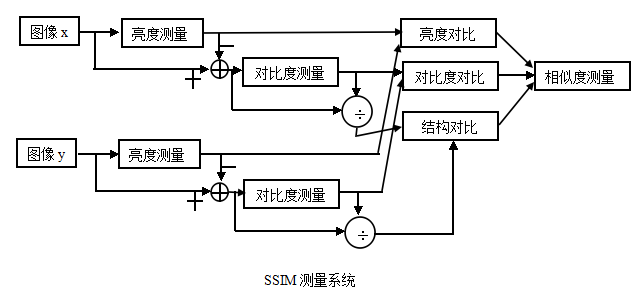基本流程为：

（1）对于输入的x和y，首先计算出（亮度测量）luminance measurement，进行比对，得到第一个相似性有关的评价；
（2）再减去luminance的影响，计算（对比度测量）contrast measurement，比对，得到第二个评价；
（3）再用上一步的结果除掉对比度的影响，再进行structure的比对。最后将结果combine，得到最终的评价结果。

从实现角度来讲，
（1）亮度luminance用均值表征，以标准图像x为例，当然还有另外一张作为对比的图像y,公式是一样的，如下：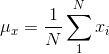（2）对比度contrast用经过均值归一化之后的方差表征，以标准图像x为例，当然还有另外一张作为对比的图像y,公式是一样的如下：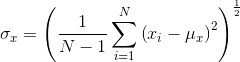（3）结构用相关系数（就是统计意义上的 r ，协方差与方差乘积的比值）。
相关系数本来的公式如下：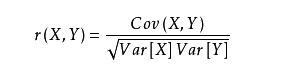在这里即为：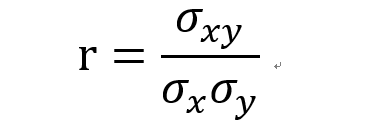其中，有：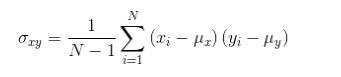注意：

上面的计算我们在实际应用的时候一般不这样去逐像素计算，一般采用高斯核函数（即高斯卷积）计算图像的均值、方差以及协方差，而不是采用遍历像素点的方式，以换来更高的效率。

具体的计算公式如下所示：
（1）亮度luminance测量值——实际上式ux，uy的函数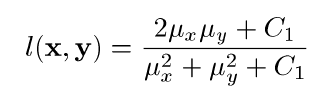（2）对比度contrast测量值——实际上是δx和δy的函数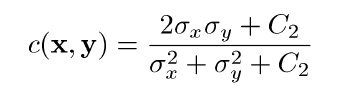（3）结构structure测量值——实际上是δxy与δx，δy的函数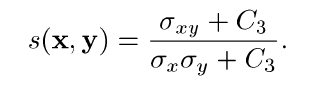其中，有：最终的结构相似性公式如下：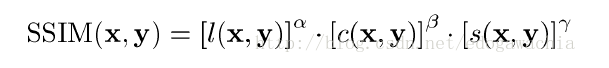其中三个参数α，β，γ 用来表示这三个模块的重要性。
其中SSIM表示相似度，这个算子应当满足作为度量的基本性质，即

Symmetry，交换x和y顺序不影响结果。Boundedness，值要有界，这里时小于等于1.。Unique maximum，最大值，即1，只有当 x = y 时候取到。

为了得到简化形式，令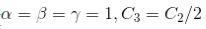于是得到下面的简化公式：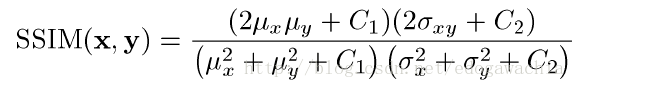这就是一般我们计算的表达式。

所以结构相似度指数从图像组成的角度将结构信息定义为独立于亮度、对比度的反映场景中物体结构的属性，并将失真建模为亮度、对比度和结构三个不同因素的组合。用均值作为亮度的估计，标准差作为对比度的估计，协方差作为结构相似程度的度量。

补充：
但是由于SSIM应该应用于局部，这是为了拟合人类视觉的局部性的特点，因此实际上我们用 mean-SSIM或者MSSIM，对各个local window的SSIM求平均。什么意思呢？
实际上就是先将一副图像划分成一些区域，对每一个区域求一个SSIM的值，然后一幅图像上面的所有区域的SSIM的平均值作为整个图像的SSIM值。

三、SSIM的实现
（1）方式一：python的skimage库中实现了一些常见的评价指标如
from skimage.measure import compare_mse     #均方误差
from skimage.measure import compare_psnr    #峰值信噪比
from skimage.measure import compare_ssmi    #结构相似性
（2）自己实现：代码如下


展开全文图像质量评价指标
• SSIM一、结构相似性二、SSIM指数2.1 亮度对比函数2.2 对比度对比函数2.3 结构对比函数2.4 SSIM测量函数2.5 SSIM函数满足的三个条件三、MSSIM四、实现 一、结构相似性 自然图像具有极高的结构性，表现在图像的像素间...


SSIM
一、结构相似性二、SSIM指数2.1 亮度对比函数2.2 对比度对比函数2.3 结构对比函数2.4 SSIM测量函数2.5 SSIM函数满足的三个条件
三、MSSIM四、实现

一、结构相似性
自然图像具有极高的结构性，表现在图像的像素间存在着很强的相关性，这些相关性在视觉场景中携带着关于物体结构的重要信息。我们假设人类视觉系统（HSV）主要从可视区域内获取结构信息。所以通过探测结构信息是否改变来感知图像失真的近似信息，衡量两幅图像的相似度
二、SSIM指数
物体表面的亮度信息与照度和反射系数有关，且场景中的物体的结构与照度是独立的，反射系数与物体有关。我们可以通过分离照度对物体的影响来探索一张图像中的结构信息。这里，把与物体结构相关的亮度和对比度作为图像中结构信息的定义。因为一个场景中的亮度和对比度总是在变化的，所以我们可以通过分别对局部的处理来得到更精确的结果。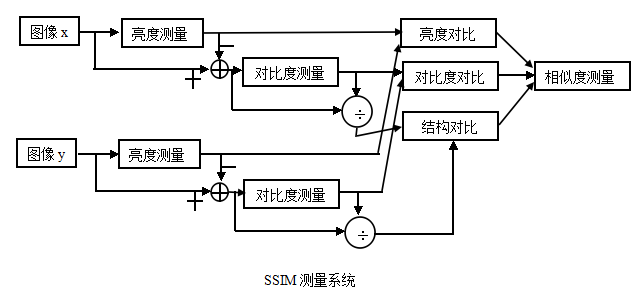SSIM测量系统由三个对比模块组成：亮度、对比度、结构
2.1 亮度对比函数
将图像的平均灰度作为亮度测量的估计

μ

X

=

1

H

×

M

∑

i

=

1

H

∑

j

=

1

M

X

(

i

,

j

)

\mu_X = \frac{1}{H\times M} \sum\limits_ {i=1}^{H}\sum\limits_{j=1}^{M} X(i, j)

于是两幅图像的亮度对比函数

l

(

x

,

y

)

=

2

μ

x

μ

y

+

C

1

μ

x

2

+

μ

y

2

+

C

1

l(x, y) = \frac{2\mu_x\mu_y+C_1}{\mu_x^2+\mu_y^2+C_1}

2.2 对比度对比函数
将图像的标准差作为对比度测量估计

σ

X

=

(

1

H

+

W

−

1

∑

i

=

1

H

∑

j

=

1

M

(

X

(

i

,

j

)

−

μ

X

)

2

)

1

2

\sigma_X = (\frac{1}{H+W-1} \sum\limits_ {i=1}^{H}\sum\limits_{j=1}^{M} (X(i, j)-\mu_X)^2)^{\frac{1}{2}}

于是两幅图像的对比度对比函数

c

(

x

,

y

)

=

2

σ

x

σ

y

+

C

2

σ

x

2

+

σ

y

2

+

C

2

c(x, y) = \frac{2\sigma_x\sigma_y+C_2}{\sigma_x^2+\sigma_y^2+C_2}

2.3 结构对比函数
结构对比函数

s

(

x

,

y

)

=

σ

x

y

+

C

3

σ

x

σ

y

+

C

3

s(x,y) = \frac{\sigma_{xy}+C_3}{\sigma_x\sigma_y+C_3}

2.4 SSIM测量函数
最后， 以上三个对比函数构成了SSIM函数

S

S

I

M

(

x

,

y

)

=

f

(

l

(

x

,

y

)

,

c

(

x

,

y

)

,

s

(

x

,

y

)

)

=

[

l

(

x

,

y

)

]

α

[

c

(

x

,

y

)

]

β

[

s

(

x

,

y

)

]

γ

\begin{aligned} SSIM(x, y) &= f(l(x, y), c(x, y), s(x, y))\\ &= [l(x, y)]^{\alpha}[c(x, y)]^\beta [s(x, y)]^\gamma\\ \end{aligned}

其中，

α

、

β

、

γ

>

0

\alpha 、\beta、\gamma >0

，用来调整这三个模块的重要性 假设

α

、

β

、

γ

都

为

1

\alpha 、\beta、\gamma 都为1

，

C

3

=

C

2

/

2

C_3 = C_2/2

,则

S

S

I

M

(

x

,

y

)

=

(

2

μ

x

μ

y

+

C

1

)

(

2

σ

x

y

+

C

2

)

(

μ

x

2

+

μ

y

2

+

C

1

)

(

σ

x

2

+

σ

y

2

+

C

2

)

SSIM(x, y) = \frac{(2\mu_x\mu_y+C_1)(2\sigma_{xy}+C_2)}{(\mu_x^2+\mu_y^2+C_1)(\sigma_x^2+\sigma_y^2+C_2)}

SSIM函数的值域为[0, 1], 值越大说明图像失真越小，两幅图像越相似
2.5 SSIM函数满足的三个条件
对称性：

S

(

x

,

y

)

=

S

(

y

,

x

)

S(x, y) = S(y, x)

有界性：

S

(

x

,

y

)

≤

1

S(x, y) \le 1

最大值唯一性：当且仅当x=y时，

S

(

x

,

y

)

=

1

S(x, y) = 1

三、MSSIM
在图像质量评估之中，局部求SSIM指数的效果要好于全局。第一，图像的统计特征通常在空间中分布不均；第二，图像的失真情况在空间中也是变化的；第三，在正常视距内，人们只能将视线聚焦在图像的一个区域内，所以局部处理更符合人类视觉系统的特点；第四，局部质量检测能得到图片空间质量变化的映射矩阵，结果可服务到其他应用中。
可以利用滑动窗将图像分块，令分块总数为N，考虑到窗口形状对分块的影响，采用高斯加权计算每一窗口的均值、方差以及协方差，然后计算对应块的结构相似度SSIM，最后将平均值作为两图像的结构相似性度量，即平均结构相似性MSSIM：
四、实现
import torch
import torch.nn.functional as F
from math import exp
import numpy as np

# 创建一维高斯分布向量
def gaussian(window_size, sigma):
gauss = torch.Tensor([exp(-(x-window_size//2)**2)/float(2*sigma**2) for x in range(window_size)])
return gauss/gauss.sum()

# 创建高斯核
def create_window(window_size, channel=1):
# unqueeze(1) 在第二维上增加一个维度
_1D_window = gaussian(window_size, 1.5).unsqueeze(1)
# t() 转置
_2D_window = _1D_window.mm(_1D_window.t()).float().unsqueeze(0).unsqueeze(0)
window = _2D_window.expand(channel, 1, window_size, window_size).contiguous()
return window

# 计算ssim
# 采用归一化的高斯核来 代替计算像素的均值
def ssim(img1, img2, window, window_size, channel=1, size_average=True):

(
mu1 = F.conv2d(img1, window, padding=window_size//2, stride=1, groups=channel)
mu2 = F.conv2d(img2, window, padding=window_size//2, stride=1, groups=channel)

mu1_sq = mu1.pow(2)
mu2_sq = mu2.pow(2)
mu1_mu2 = mu1 *mu2

sigma1_sq = F.conv2d(img1*img1, window, padding=window_size//2, stride=1, groups=channel) - mu1_sq
sigma2_sq = F.conv2d(img2*img2, window, padding=window_size//2, stride=1, groups=channel) - mu2_sq
sigma_12 = F.conv2d(img1*img2, window, padding=window_size//2, stride=1, groups=channel) - mu1_mu2

c1 = 0.01 **2
c2 = 0.03 **2

ssim_map = (2*(mu1_mu2 +c1)*(2*sigma_12 + c2)) / ((mu1_sq + mu2 + c1)*(sigma1_sq + sigma2_sq + c2))

if size_average:
return ssim_map.mean()
else:
return ssim_map.mean(1).mean(1).mean(1)

class SSIM(torch.nn.Module):
def __init__(self, window_size=11, channel, size_average=True):
super(SSIM, self).__init__()
self.window_size = window_size
self.size_average = size_average
self.channel = channel
self.window = create_window(window_size, channel)

def forward(self, img1, img2):
(_, channel, _, _) = img1.size()

if channel == self.channel and self.window.data.type() == img1.data.type():
window = self.window
else:
window = create_window(self.window_size, channel)
if img1.is_cuda:
window.cuda(img1.get_device())
window = window.type_as(img1)
self.window = window
self.channel = channel

return ssim(img1, img2, self.window, self.window_size, channel, self.size_average)

展开全文计算机视觉
• 与原始语料库和结构化本体的联合语义相似性评估，用于面向语义的服务发现研究论文
• SSIM(structural similarityindex)，结构相似性，是一种衡量两幅图像相似度的指标。该指标首先由德州大学奥斯丁分校的图像和视频工程实验室(Laboratory for Image and Video Engineering)提出。SSIM使用的两张图像...
一、SSIM算法简介
SSIM(structural similarity index)，结构相似性，是一种衡量两幅图像相似度的指标。该指标首先由德州大学奥斯丁分校的图像和视频工程实验室(Laboratory for Image and Video Engineering)提出。SSIM使用的两张图像中，一张为未经压缩的无失真图像，另一张为失真后的图像。 标题为：Image Quality Assessment: From Error Visibility to Structural Similarity 地址为：https://ieeexplore.ieee.org/stamp/stamp.jsp?tp=&arnumber=1284395
与PSNR一样，SSIM也经常用作图像质量的评价。
二、SSIM算法原理
对SSIM算法，其输入是两张图片，即要求结构相似性的两张图片。其中一张是未经压缩的无失真图像(即ground truth)，另一张就是需要与无失真图片对比的图片。物体表面的亮度信息与照度和反射系数有关，且场景中的物体的结构与照度是独立的，反射系数与物体有关。我们可以通过分离照度对物体的影响来探索一张图像中的结构信息。这里，把与物体结构相关的亮度和对比度作为图像中结构信息的定义。因为一个场景中的亮度和对比度总是在变化的，所以我们可以通过分别对局部的处理来得到更精确的结果。
算法处理的原理图如下图所示：由SSIM测量系统可得相似度的测量可由三种对比模块组成，分别为：亮度，对比度，结构。接下来我们将会对这三模块函数进行定义。
首先，对于离散信号，我们以平均灰度来作为亮度测量的估计：(1)
亮度对比函数l（x,y）是关于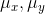的函数。
然后，由测量系统知道要把平均灰度值从信号中去除，对于离散信号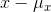，可使用标准差来做对比度估量值。（2）
对比度对比函数c(x,y)就是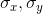的函数。
接下来，信号被自己的标准差相除，结构对比函数就被定义成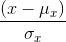和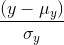的函数。
最后，三个对比模块组合成一个完整的相似测量函数：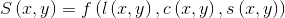（3）

S（x,y）应该满足以下三个条件：
（1） 对称性：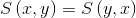；
（2） 有界性：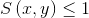；
（3） 最大值唯一性：当且仅当x=y时，S（x,y）=1 。

现在，我们定义三个对比函数。
亮度对比函数：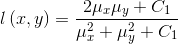（4）
常数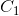是为了避免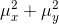接近0时造成系统的不稳定。
特别的，我们选择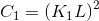，L为图像灰度级数，对于8-bit灰度图像，L=255，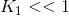。公式（4）满足上述三个条件。
对比度对比函数：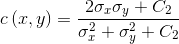（5）
常数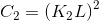，且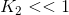。公式（5）依然满足上述三个条件。
结构对比函数：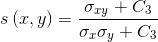（6）
其中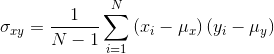（7）
最后把三个函数组合起来，得到SSIM指数函数：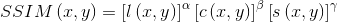（8）
这里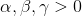，用来调整三个模块间的重要性。
为了得到简化形式，设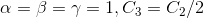，得到：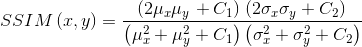（9）
三、SSIM指数应用于图像质量评估
在图像质量评估之中，局部求SSIM指数的效果要好于全局。第一，图像的统计特征通常在空间中分布不均；第二，图像的失真情况在空间中也是变化的；第三，在正常视距内，人们只能将视线聚焦在图像的一个区域内，所以局部处理更符合人类视觉系统的特点；第四，局部质量检测能得到图片空间质量变化的映射矩阵，结果可服务到其他应用中。
所以，在上述公式中，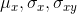都加入了一个8*8的方形窗，并且逐像素的遍历整幅图片。每一步计算，和SSIM都是基于窗口内像素的，最终得到一个SSIM指数映射矩阵，由局部SSIM指数组成。然而，简单的加窗会使映射矩阵出现不良的“分块”效应。为解决这问题，我们使用11*11的对称高斯加权函数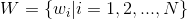作为加权窗口，标准差为1.5，且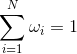（10）

则的估计值表示为：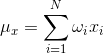（11）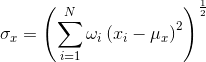（12）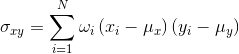（13）
应用这种加窗方法，映射矩阵就可展现出局部各向同性的性质。
在这里，经过一些实验总结，我们把K1设为0.01，K2设为0.03，然后用平均SSIM指数作为整幅图像的估计质量评价：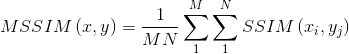（14）
其中X,Y为图像，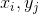为局部SSIM指数在映射中的位置，MN为局部窗口的数量。
三、python代码实现
代码是从项目里面直接贴过来的，只改了其中一些部分，代码的目录格式如下所示：
├─python-ssim │  │  python-ssim.py │  │  README.md │  │  使用.txt │  └─data │          1.jpg │          2.jpg │          3.jpg │          4.jpg │          5.png │          6.png
python-ssim.py文件
import numpy
import scipy.ndimage
from numpy.ma.core import exp
from scipy.constants.constants import pi

'''
The function to compute SSIM
@param param: img_mat_1 1st 2D matrix
@param param: img_mat_2 2nd 2D matrix
'''
def compute_ssim(img_mat_1, img_mat_2):
#Variables for Gaussian kernel definition
gaussian_kernel_sigma=1.5
gaussian_kernel_width=11
gaussian_kernel=numpy.zeros((gaussian_kernel_width,gaussian_kernel_width))

#Fill Gaussian kernel
for i in range(gaussian_kernel_width):
for j in range(gaussian_kernel_width):
gaussian_kernel[i,j]=\
(1/(2*pi*(gaussian_kernel_sigma**2)))*\
exp(-(((i-5)**2)+((j-5)**2))/(2*(gaussian_kernel_sigma**2)))

#Convert image matrices to double precision (like in the Matlab version)
img_mat_1=img_mat_1.astype(numpy.float)
img_mat_2=img_mat_2.astype(numpy.float)

#Squares of input matrices
img_mat_1_sq=img_mat_1**2
img_mat_2_sq=img_mat_2**2
img_mat_12=img_mat_1*img_mat_2

#Means obtained by Gaussian filtering of inputs
img_mat_mu_1=scipy.ndimage.filters.convolve(img_mat_1,gaussian_kernel)
img_mat_mu_2=scipy.ndimage.filters.convolve(img_mat_2,gaussian_kernel)

#Squares of means
img_mat_mu_1_sq=img_mat_mu_1**2
img_mat_mu_2_sq=img_mat_mu_2**2
img_mat_mu_12=img_mat_mu_1*img_mat_mu_2

#Variances obtained by Gaussian filtering of inputs' squares
img_mat_sigma_1_sq=scipy.ndimage.filters.convolve(img_mat_1_sq,gaussian_kernel)
img_mat_sigma_2_sq=scipy.ndimage.filters.convolve(img_mat_2_sq,gaussian_kernel)

#Covariance
img_mat_sigma_12=scipy.ndimage.filters.convolve(img_mat_12,gaussian_kernel)

#Centered squares of variances
img_mat_sigma_1_sq=img_mat_sigma_1_sq-img_mat_mu_1_sq
img_mat_sigma_2_sq=img_mat_sigma_2_sq-img_mat_mu_2_sq
img_mat_sigma_12=img_mat_sigma_12-img_mat_mu_12;

#c1/c2 constants
#First use: manual fitting
c_1=6.5025
c_2=58.5225

#Second use: change k1,k2 & c1,c2 depend on L (width of color map)
l=255
k_1=0.01
c_1=(k_1*l)**2
k_2=0.03
c_2=(k_2*l)**2

#Numerator of SSIM
num_ssim=(2*img_mat_mu_12+c_1)*(2*img_mat_sigma_12+c_2)
#Denominator of SSIM
den_ssim=(img_mat_mu_1_sq+img_mat_mu_2_sq+c_1)*\
(img_mat_sigma_1_sq+img_mat_sigma_2_sq+c_2)
#SSIM
ssim_map=num_ssim/den_ssim
index=numpy.average(ssim_map)

print(index)

return index

compute_ssim(img_mat_1, img_mat_2)

三、结果示例
示例1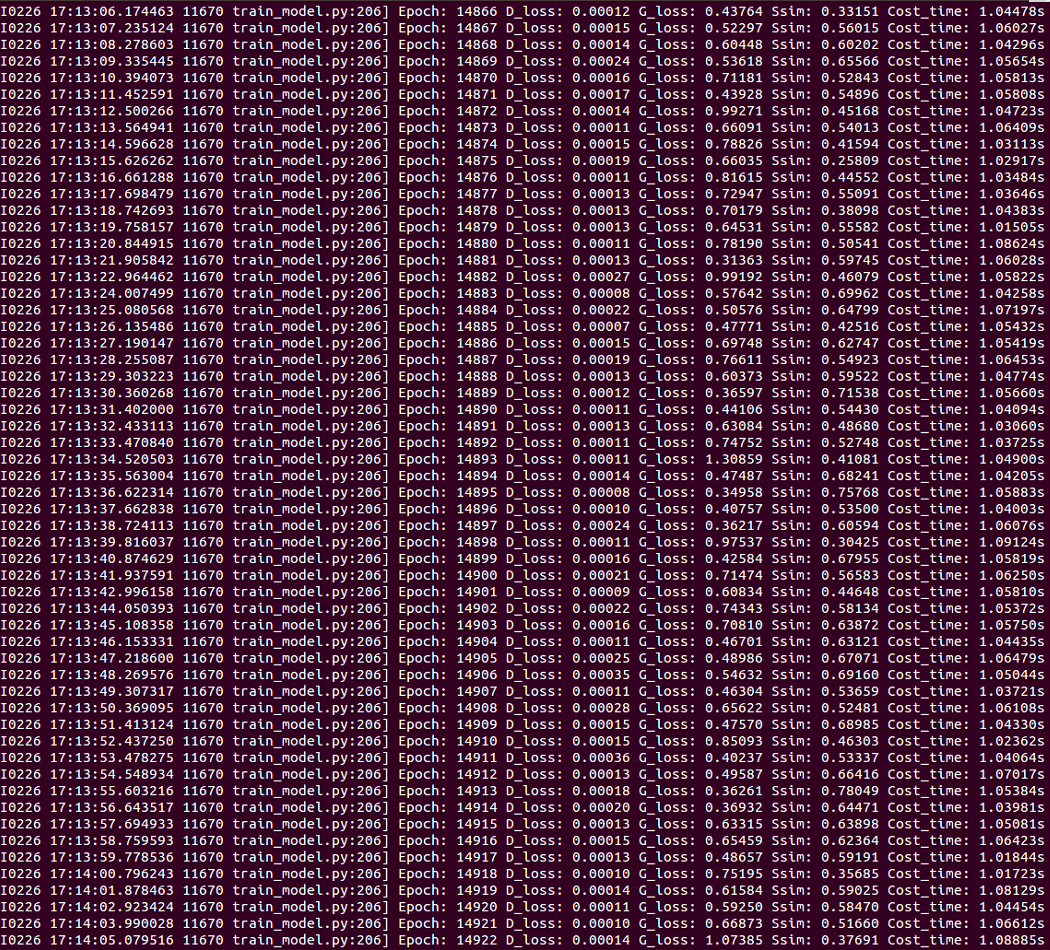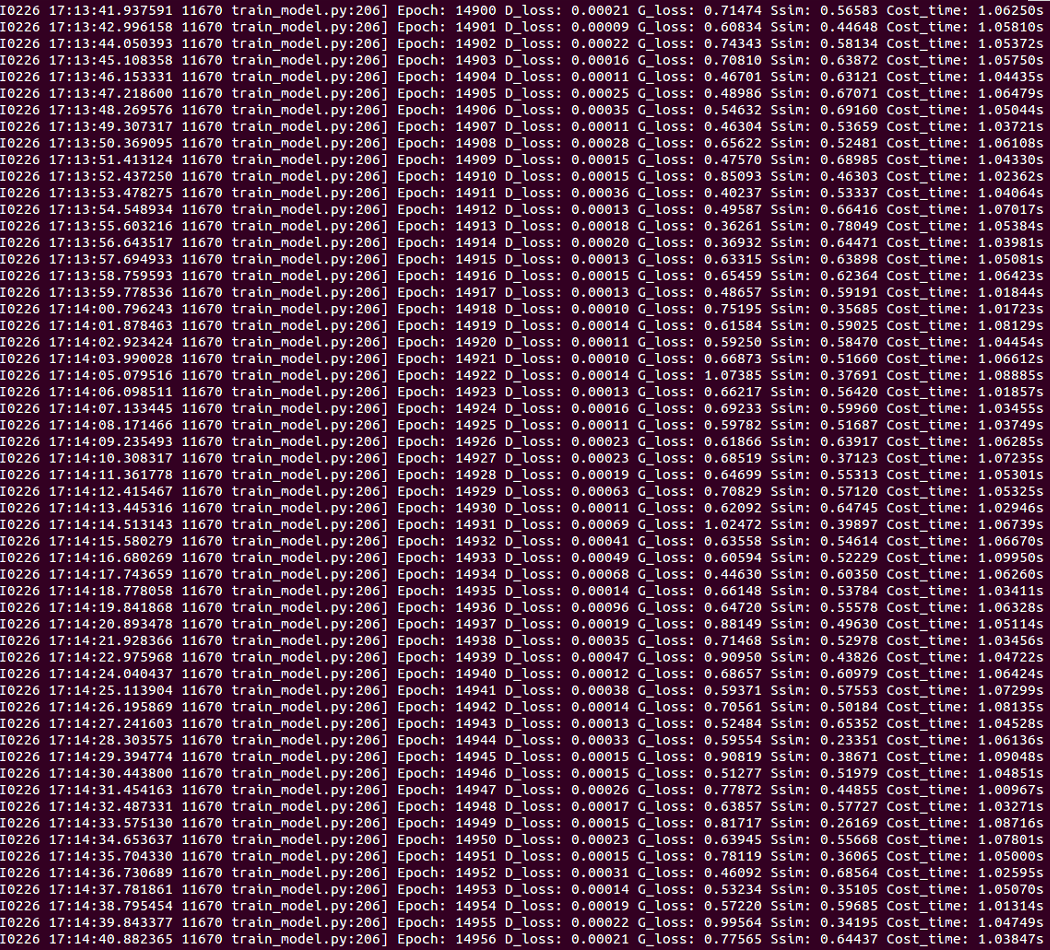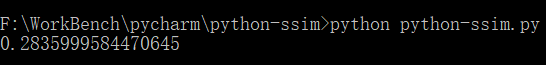示例2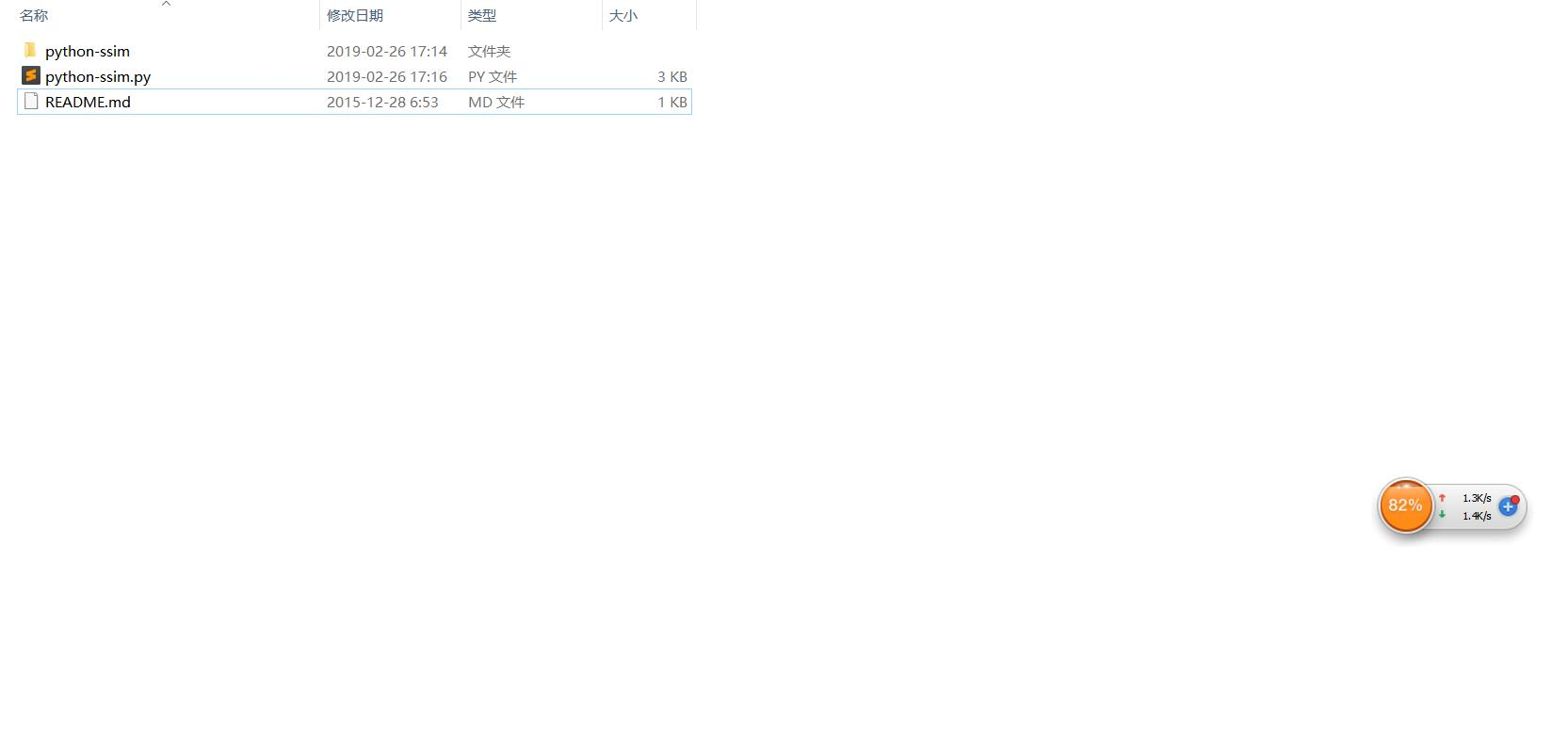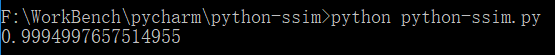示例3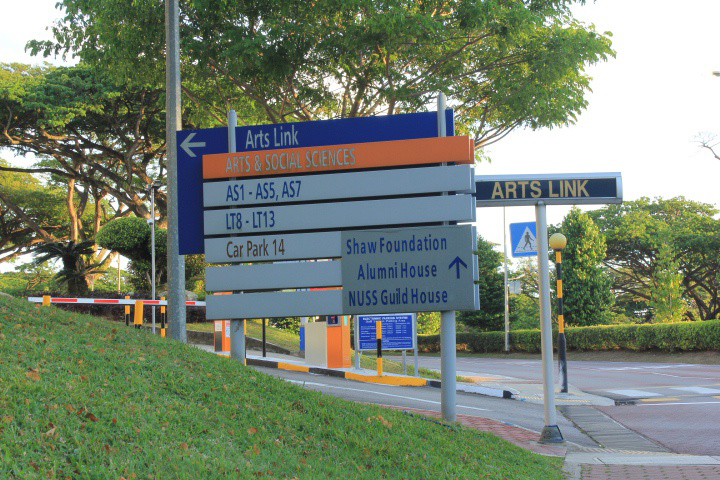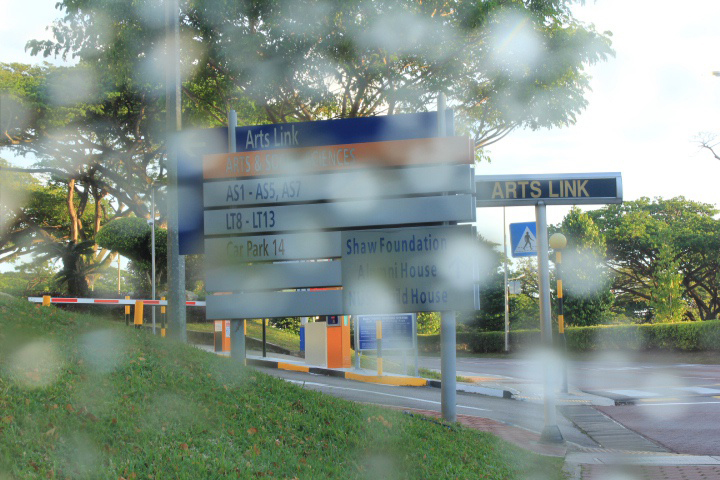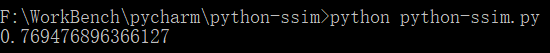参考：https://blog.csdn.net/ecnu18918079120/article/details/60149864SSIM指数应用于图像质量评估
https://blog.csdn.net/leviopku/article/details/84635897
展开全文SSIM
• 大型化合物数据库的多样性评估和比较需要在可承受的时间内计算数百万种化合物的相似性。 ParaSim通过根据一台计算机上可用的计算内核数量并行化计算来解决这一挑战。 针对大量查询结构相对于大量引用结构的吞吐量...开源软件
• 一、结构相似性（structural similarity）  自然图像具有极高的结构性，表现在图像的像素间存在着很强的相关性，尤其是在空间相似的情况下。这些相关性在视觉场景中携带着关于物体结构的重要信息。我们假设人类视觉...图像处理 质量评价 SSIM matlab
• 针对现有图像质量评估方法不能有效评价所有失真类型图像、计算量大等问题进行了研究，受人眼视觉特性的启发，提出了一种新的基于图像边缘结构相似性的全参考质量评价方法。该方法利用图像边缘梯度对图像失真的敏感性...图像质量评价 全参考 Prewitt算子
• 平均结构相似性MSSIM (Mean Structural SIMilarity)表明图像分割结果与参考图像的平均局部结构相似性，其取值也在0到1之间，取值越大表明分割质量越好，当MSSIM=1时，对应图像分割结果与参考图像完全相同。
• 其中一个是PSNR(峰值性噪比)，还一个便是SSIM（结构相似性评价）。由于最近有用到SSIM，自己写了个python代码版本的SSIM放在文章最后。 SSIM算法简单介绍 其中ux、uy分别表示图像X和Y的均值，σX、σY分别表示...SSIM
• 基于梯度的结构相似度(GSSIM)： SSIM 算法不能较好地评价严重模糊图像的质量，有鉴于此提出GSSIM。主要是SSIM中结构信息在模糊情况，不能代表结构信息，所以对图像进行梯度计算，得到图像的边缘，然后再对图像结构...图像质量 图像失真 图像评价
• 当评估分子相似性时，经常...Fraggle就是一种特殊的相似性评估算法。 Fraggle与现有相似度评估方法之间的差异 Fraggle是用于考虑GSK中分子相似性的方法之一，最初是在2008年使用Daylight工具包实现的。RDKit...
• ## 序列的相似性

千次阅读 2019-05-04 08:02:20
序列的相似性可以是定量的数值，也可以是定性的描述。相似度是一个数值，反映两条序列的相似程度。关于两条序列之间的关系，有许多名词，如相同、相似、同源、同功、直向同源、共生同源等。在进行序列比较时经常使用...PAM矩阵 基因序列
• 结构相似形特征是图像全参考评价(FR-IQA)中经典的一个...一、结构相似性（structural similarity） 自然图像具有极高的结构性，表现在图像的像素间存在着很强的相关性，尤其是在空间相似的情况下。这些相关性在视...
• 相似性和相异性（dissimilarity）是根据数据对象的属性值评估的，通常涉及到距离度量。相似性（similarity）和相异性（dissimilarity）是负相关的，统称为临近性（proximity）。 在聚类分析中，聚类算法的第一步都是...
• 梯度幅相似性偏差 GMSD（以下使用GMSD作为简称）的提出主要是受到图像梯度场对于图像退化比较敏感这一事实的启发提出的。GMSD出现比较晚（2013年），在此之前有很多比较流行的评估指标比如VIF和FSIM等等，但是在当时...GMSD
• 在本文中，我们提出了 Tiresias，这是一种基于相似性的大规模框架，可通过链接预测来预测 DDI。 Tiresias 将各种来源的毒品相关数据和知识作为输入，并提供 DDI 预测作为输出。 该过程从输入数据的语义整合开始，...
• 介绍并讨论了有代表的三种评估方法：SAAM，ATAM，ALPSM，在此基础上提出一个概念上的比较框架来分析这些评估方法的相似与差异，并进一步对方法的结合、重用，以及在设计中引入评估及实践中的使用等问题作了探讨。质量属性
• 传统全参考图像质量衡量标准 结构相似度 结构相似度SSIM 是目前最为成功、使用范围最广泛的评价标准，在图像科学的很多领域都是必备的评价指标，如果对于场景的把握不是非常熟悉使用ssim是一个推荐选项 ssim的设计...SSIM
• 相似性评估 化合物的指纹对于使用计算机考虑化合物的相似性是必需的。已经提出了各种评估方法，但是最常用的评估方法称为“Tanimoto系数”。使用以下等式从两个分子A和B的位阵列指纹计算Tanimoto系数： 导入库...化学信息学 分子指纹 Python
• ## 测试开发需要学习的知识结构

万次阅读 多人点赞 2018-04-12 10:40:58
– 测试内部数据结构的有效，等。 具体包含的逻辑覆盖有： – 语句覆盖 – 判定覆盖 – 条件覆盖 – 判定－条件覆盖 – 条件组合覆盖 – 路径覆盖。 3、白盒测试&黑盒测试对比 白盒测试技术 (White Box Testing) ...测试开发
• 从这一观点出发，提出了关于相似性判断的不对称结构的心理学研究成果，并尝试了在商标法中表达这种心理行为时要考虑的因素，相似性判断的不对称性是一个重要的因素。商标的相似性是由商标的上下文问题，商标的吸引...
• 它侧重于体系结构评估的时间可用以及所研究体系结构的质量要求。此步骤中的体系结构演示非常重要，因为它会影响分析的质量。本演讲中涉及的一些关键问题是：技术约束，与正在评估的系统交互的其他系统，以及为...
• 7.1.1 评估关注的质量属性　软件体系结构的设计是整个软件开发过程中关键的一步。对于当今世界上庞大而复杂的系统来说，如果没有一个合适的体系结构而要有一个成功的软件设计几乎是不可想象的。 不同类型的系统需要...
• 为了解决这个问题，本文提出了一种基于两阶段低级特征的多尺度结构图像质量评估，通过结合观察条件和分辨率的变化，该评估比以前的单尺度方法更具灵活。 在此多尺度框架中，将不同的权重分配给具有不同重要级别...研究论文...